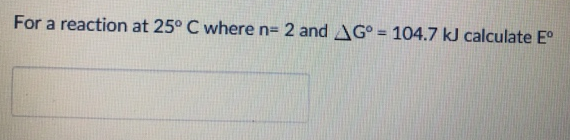# Problem: For a reaction at 25°C where n = 2 and ΔG° = 104.7 kJ calculate E°

###### FREE Expert Solution
85% (54 ratings)###### Problem Details

For a reaction at 25°C where n = 2 and ΔG° = 104.7 kJ calculate E°